# LeetCode Weekly Contest 115

https://leetcode.com/contest/weekly-contest-115

## Check Completeness of a Binary Tree

Given a binary tree, determine if it is a complete binary tree.

Definition of a complete binary tree from Wikipedia:
In a complete binary tree every level, except possibly the last, is completely filled, and all nodes in the last level are as far left as possible. It can have between $1$ and $2^h$ nodes inclusive at the last level h.

Example 1: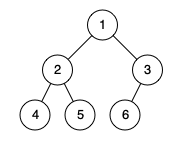Example 2: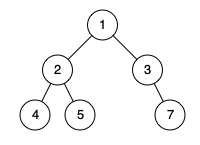Note:

1. The tree will have between 1 and 100 nodes.

BFS把二叉树写成数组形式（就是input的那种形式），检查中间是否含有null即可:

## Prison Cells After N Days

There are 8 prison cells in a row, and each cell is either occupied or vacant.

Each day, whether the cell is occupied or vacant changes according to the following rules:

If a cell has two adjacent neighbors that are both occupied or both vacant, then the cell becomes occupied.
Otherwise, it becomes vacant.
(Note that because the prison is a row, the first and the last cells in the row can’t have two adjacent neighbors.)

We describe the current state of the prison in the following way: cells[i] == 1 if the i-th cell is occupied, else cells[i] == 0.

Given the initial state of the prison, return the state of the prison after N days (and N such changes described above.)

Example 1:

Example 2:

Note:

1. cells.length == 8
2. cells[i] is in {0, 1}
3. 1 <= N <= 10^9

i位的取值取决于i-1位和i+1位是否相同，而首位和末尾除了第0天都会是0。

cells的变化以14天为一个周期，所以只需要看它对应这14天中的哪一天就可以了。当模14结果为0时对应第14天，其他则对应相应的模14余数。

## Regions Cut By Slashes

In a N x N grid composed of 1 x 1 squares, each 1 x 1 square consists of a /, \, or blank space. These characters divide the square into contiguous regions.

(Note that backslash characters are escaped, so a \ is represented as "\\".)

Return the number of regions.

Example 1:Example 2: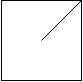Example 3: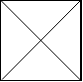Example 4: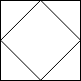Example 5: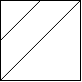Note:

1. 1 <= grid.length == grid.length <= 30
2. grid[i][j] is either '/', '\', or ' '.## Delete Columns to Make Sorted III

We are given an array A of N lowercase letter strings, all of the same length.

Now, we may choose any set of deletion indices, and for each string, we delete all the characters in those indices.

For example, if we have an array A = ["babca","bbazb"] and deletion indices {0, 1, 4}, then the final array after deletions is ["bc","az"].

Suppose we chose a set of deletion indices D such that after deletions, the final array has every element (row) in lexicographic order.

For clarity, A is in lexicographic order (ie. A <= A <= ... <= A[A.length - 1]), A is in lexicographic order (ie. A <= A <= ... <= A[A.length - 1]), and so on.

Return the minimum possible value of D.length.

Example 1:

Example 2:

Example 3:

Note:

1. 1 <= A.length <= 100
2. 1 <= A[i].length <= 100